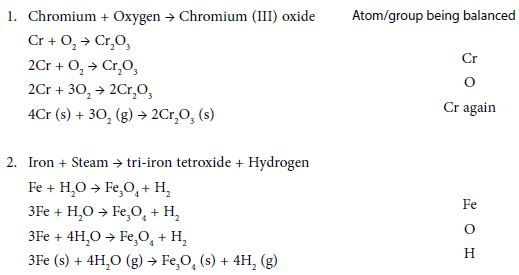# Balancing Chemical Equations

Write the correct formula for the reactants and products; reactants on left of arrow, products on right. Choose the most complicated compound that contains the greatest number of atoms, whether it is reactant or product. Start with the element in that compound that has the greatest number of atoms (not H or O or a polyatomic ion such as sulphate, nitrate, carbonate etc.). Balance the number of atoms in this compound with the corresponding atom on the other side by putting the appropriate numbers before the formulae. Repeat for other atoms. Next balance any polyatomic groups (such as SO4, NO3 and CO3) that appear on both sides of the equation. Finally check the numerical coefficients (the numbers before the formulae) to ensure they are whole numbers and in the lowest possible ratio. Include state symbols if required, in brackets, after the formulae.

E.g. Na(s), H2O (1), N2 (g), Cue2+ (aq)

Remember: it is not always possible to follow the guidelines in the previous slides exactly. Never change the formulae, only the numbers before the formulae (the coefficients). See examples below: